## 5.8Accelerating convergence

The Gauss-Seidel method sweeps across cells, calculating the changes in values of ﬁelds, e.g.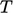. Each sweep propagates the changes inthrough cells in the domain in much the same way that the ﬂow transportsphysically.

The method is therefore remarkably eﬀective, so the scope to accelerate convergence to reduce computational cost is limited. Two options that may reduce cost, relating to mesh numbering, are described in this section.

### Symmetric Gauss-Seidel

The Gauss-Seidel method sweeps through equations in sequence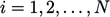of cell numbering. The changes in the solution “ﬂow” in a direction, based on the choice of cell numbering which may be arbitrary and therefore not optimal.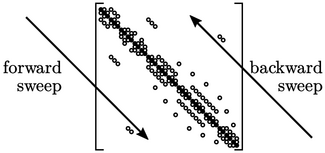The symmetric Gauss-Seidel method follows the standard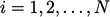sequence initially, known as a forward sweep. For every second sweep, the sequence is executed in reverse, i.e.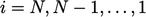, known as a backward sweep.

The change in sweep direction moderates the directional bias of the standard Gauss-Seidel method which may reduce the total iterations for convergence and reduce cost.

### Mesh numbering

When a matrix equation is solved in sequence, values from one row of the equation are substituted in subsequent equations. Convergence improves if the substitutions are made quickly, e.g. in the subsequent 2 or 3 equations rather than in the last equation.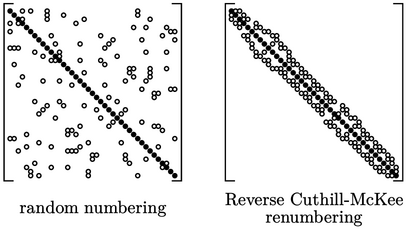Convergence is therefore aﬀected by the cell numbering which determines the position of non-zero coeﬃcients in the matrix. If the numbering is approaching random, e.g. as a consequence of the mesh generation, convergence is sub-optimal.

Where this occurs, a method such as reverse Cuthill-McKee2 can be applied to renumber the cells of a mesh to cluster non-zero coeﬃcients around the diagonal, as shows above.

The matrix in Sec. 5.1 is from a mesh generated in a structured manner with ordered indexing along rows of cells. Renumbering a matrix like that generally provides little beneﬁt since it already has many non-zero coeﬃcients clustered around the diagonal.

2Elizabeth Cuthill and James McKee, Reducing the bandwidth of sparse symmetric matrices, 1969; “reversed” by Alan George and Joseph Liu, Computer Solution of Large Sparse Positive Deﬁnite Systems, 1981.

Notes on CFD: General Principles - 5.8 Accelerating convergence